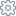Sunday December 3, 2023, 4:18 pm

# 2 - Setting the Metric Units• Step 1
• Help
• FAQ
• To set the units of measurement you use the menu command SettingsMetric Units, or the tooland then selecting the Metrics tab.
• Choose the unit of measure and set the accuracy of the input data with the scale factor. For example, if the accuracy is millimeter, you can use mm 1x, or cm 10x or m 1000x.
• Confirm the changes by pressing the Apply button and close the dialog.

All sizes are always integer numbers without decimal separator.Let's consider for example a box with sides 12.7 cm, 14 cm and 23.2 cm.

Although the units employed are centimeters, the actual accuracy is millimeters and the program input must be 127, 140, and 232.

Output, which follows a more conventional formatting, depends on the settings used, as shown in the table

 User data input output output output mm 1x cm 10x m 1000x 12.7 cm 127 127 mm 12.7 cm 0.127 m 14 cm 140 140 mm 14.0 cm 0.140 m 23.4 cm 234 234 mm 23.4 cm 0.234 mD Why whole numbers? Wouldn't it be enough to keep the number of decimal digits fixed?

Unfortunately data exchange makes this approach tricky. The following example, a little exaggerated, should help to understand why we made this choice.

We have a container with side of 4.70 and in an Excel file we have a box with side of 0.122. Units do not matter, they could be meters, inches, feet, etc. The program must transform the input into two-digit decimal value, so it rounds off the number to 0.12. The solver therefore estimates that 39 packages can be loaded. Actually, such a load plan is incorrect, because only 38 packages can be loaded.

One possibility would be to always round up the value. In this case the solver would calculate loading of 36 packages, which is certainly a bad estimate.

The program uses whole numbers because it never changes the input data.Sitemap